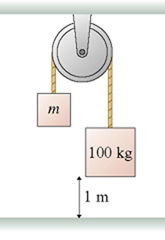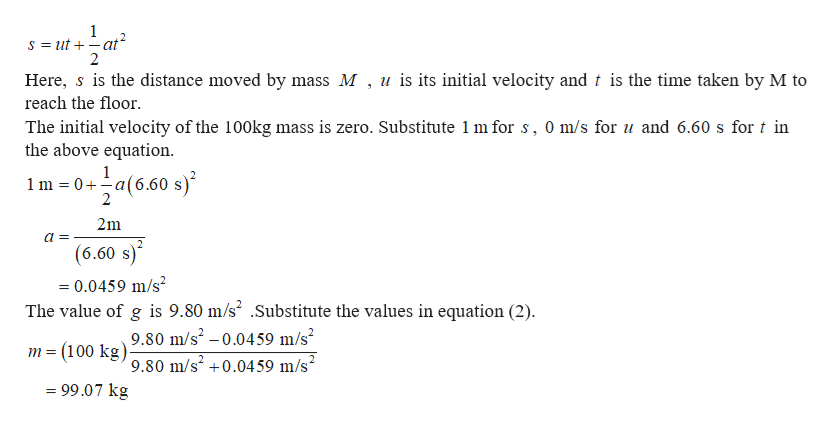100 kg1 m

Question

The 100 kg block in figure takes 6.60 s to reach the floor after being released from rest.  What is the mass of the block on the left?help_outlineImage Transcriptionclose100 kg 1 m fullscreen
Step 1

The forces on the two masses must be equal and also the acceleration with which the two masses move must be equal.

Step 2

The equation of motion of the ...help_outlineImage Transcriptionclose1 S ut at 2 Here, s is the distance moved by mass M , u is its initial velocity and t is the time taken by M to reach the floor The initial velocity of the 100kg mass is zero. Substitute 1 m for s, 0 m/s for u and 6.60 s for t in the above equation a(6.50 s 1 1 m 0 a 2m (6.60 s) =0.0459 m/s2 The value of g is 9.80 m/s2 .Substitute the values in equation (2). 9.80 m/s2 -0.0459 m/s2 = (100 kg)9.80 m/s2 +0.0459 m/s2 = 99.07 kg fullscreen

Want to see the full answer?

See Solution

Want to see this answer and more?

Our solutions are written by experts, many with advanced degrees, and available 24/7

See Solution
Tagged in

Physics